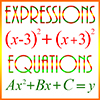# Expressions / Equations – Definitions of TermsCoefficient: The constant value in a term containing a variable. For example, 2x is a term, and 2 is the coefficient in this term. In the polynomial

\begin{align}3x^2 + 5x + 9\end{align}

3 is the coefficient of the first term, and 5 is the coefficient of the second term.

Equation: An algebraic statement that shows two algebraic expressions separated by an equals sign. This signifies that the value on the left of the equals sign is the same as the value on the right. Example: 3x – 5 = 2x + 9. This equations states that the expression on the left, 3x – 5, is equal to the expression on the right, 2x + 9. When we solve this equation, we determine that these two expressions can only be equal when x = 14.

Equivalent Equations: Two equations are equivalent if one can be transformed to the other by a simple operation, such as multiplying both sides of the equation by the same value. Example: 2x + 7 = y is equivalent to 4x + 14 = 2y, because if we multiply both sides of the first equation by 2, then we obtain the second equation. If one equation is equivalent to another, then the second equation gives us no new information that we didn’t already have in the first equation. Every solution to one equation is also a solution to the other. If two equations are not equivalent, then they are said to be independent.

Expression: A combination of algebraic terms that can be used in an equation. Example: \begin{align}x^3 + 3x – 4\end{align} An expression is not an equation, but can be used as part of an equation.

Factor: A component of a multiplication product. For example, 2 and 3 are factors of 6, since 6 = 2 x 3. Also, the factors of \begin{align}x^2 + 5x + 6\end{align} are (x + 2) and (x + 3), since (x + 2)(x + 3) = \begin{align}x^2 + 5x + 6\end{align}.

Factorization of Polynomials: An expression which shows a polynomial as a product of two or more smaller polynomials. For example, the following is a factorization of a polynomial:

\begin{align} x^2 – 5x + 6 = (x -2)(x – 3)\end{align}

The expression on the right of the equals sign is a factorization of the expression on the left.

Independent Equations: Multiple equations that are not equivalent.

Linear Equation: An equation with x, or x and y, as variables, but with every variable having an exponent of 1. Examples:

\begin{align}4x + 2 = 6\end{align}

\begin{align}5x – 3y = 17\end{align}

They are called “linear” because the graph of these equations on the coordinate plane is a straight line.

Monomial: A polynomial with only a single term. Example: \begin{align}3x^2\end{align}

Polynomial: An algebraic expression consisting of multiple terms connected by plus (+) or minus (-) signs. Example: \begin{align}x^3 + 3x – 4\end{align}

Product: The result of the multiplication of 2 or more terms in a polynomial, or two or more numbers otherwise. Example of a polynomial product:

\begin{align} (x + 2) (x – 4) = x^2 – 2x – 8\end{align}

Quadratic Equation: A polynomial equation with x as the only variable, and where the highest power of x is 2. Examples:

• \begin{align}x^2 + 4x – 5 = 0\end{align}
• \begin{align}3x^2 +2x – 15 = 0\end{align}

Quadratic Formula: This is a general formula that can be used to solve any quadratic equation.

\begin{align} x = \frac { – b \pm \sqrt {b^2 – 4ac} } {{2a}}\end{align}

where the original equation has the form:

\begin{align}ax^2 + bx + c = 0\end{align}

If the portion under the square root sign, b² – 4ac, is greater than or equal to 0, then the roots of the equation will be real numbers. Otherwise, they will be complex or imaginary numbers.

Roots of an Equation: Solutions to an equation. This term is usually used if the roots are solutions to a quadratic equation. Example: Suppose we have the equation \begin{align}x^2 – 5x + 6 = 0\end{align}. Then 2 and 3 are roots of this equation.

Simultaneous Linear Equations: Multiple linear equations in several variables, each of which has the same value in each equation. If the number of independent linear equations is the same as the number of variables or unknowns in the equations, then the values of the unknowns can be determined. Example:

2x + 6 = 4y

3x – 2 = y + 4

In this system there are 2 equations in 2 unknowns. The solution to the system of equations is x = 3 and y = 3.

Solutions to an Equation: See Roots of an Equation.

Term: A single part of a polynomial, consisting of a variable raised to a positive integer power multiplied by a constant, called a coefficient, or a single constant with no variable. A term is also called a monomial. Example:

\begin{align} 3x^2 + 5x – 9\end{align}

is a polynomial with three terms: \begin{align} 3x^2, 5x, -9\end{align} The first two terms are raised to the second and the first power, respectively. The constant term is -9, and the other two terms have coefficients of 3 and 5.

Unknown Quantity: The variable in an equation which can be solved to determine the value of the unknown. For example: In the equation 2x + 5 = 9, x is the unknown, and its value is 2.

Variable: A symbol in a polynomial, usually a letter from the end of the alphabet like x, y, or z, which may represent any number from a set, usually the set of real numbers. Example: In the polynomial

\begin{align} 3x^2 + 5x – 9\end{align}x is a variable, and 3, 5, and -9 are coefficients.

Word Problem: A problem in a “real world” situation which can be solved through the application of mathematical concepts. Examples include interest and profit problems, measurement problems, mixture problems, geometry problems, etc.